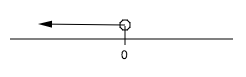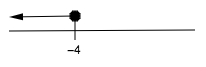### Home > CCA2 > Chapter 3 > Lesson 3.2.2 > Problem3-81

3-81.

Solve each of the following inequalities for the given variable. Represent your solutions on a number line.

1. $5+3x<5$

$x<0$2. $−3x\ge8−x$

When you divide both sides of an inequality by a negative number,
it reverses or 'flips' the sign. Here is an example of why that works:

true statement $−3<5$
multiply both sides by $−1−1·−3<5·−1$
statement is no longer true $3<−5$
reverse the inequality sign to make it true $3>−5$

This also works with division by a negative number.

Add $x$ to both sides.

$−2x\ge8$

Divide both sides by $−2$.

$x\le−4$
Did you remember to reverse the inequality sign?

$\frac{-2x}{-2}\ge \frac{8}{-2}$